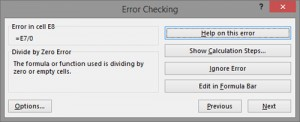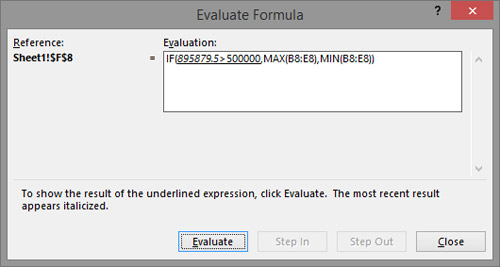# Finding errors

Errors in the calculations are a common thing. The more data in the table and the formula are the more likely we are mistaken. Excel immediately shows us an error as soon as it comes in, but it’s not always easy to find out how it came to it. Also, sometimes it may happen that we miss the error because there was no mistake in mathematical operations. Excel has several options for detecting and finding errors, which will be said in the following text …

When working in Excel, the user can encounter the following errors:

##### – A sequence of “sharps” tells us that the content exceeds the cell dimensions. It is necessary to extend the column so that the contents of the cell can be fully displayed.

#NAME? – This error usually occurs when we have incorrectly written the name of a formula that we have entered into the cell

#VALUE! – This error occurs when the formula has a wrong argument. For example, it may come up when we collate a number and a text value.

#DIV/0! – This error occurs when we divide a number by zero

#REF! – This error indicates that the formula contains a cell that is not valid. For example, it occurs when using the VLOOKUP function we try to present a value that does not exist.Finding mistakes is being done with option Error Checking which is a part of Formulas ribbon. By choosing this option we will open a dialog box which sequentially finds all errors in current worksheet. By clickning Next or Previous we’ll move to next or previous error. The Help on This Error option opens a help menu describing the type of error that is described. The Ignore Error option is used to ignore the error, and the Edit in Formula Bar option allows you to update the formula and correct the error. The Show Calculation Steps opens a dialog box for formula analysis.An analysis of the formula can be done in another way, by selecting the formula and further choosing the option Evaluate Formula in the Formulas ribbon. This option is especially important when we have complex formulas that consist of several nested formulas. By clicking the Evaluate button, we begin with the formula analysis from the left to the right, and by clicking on Step In, we analyze the ugnized formula. By clicking on Step Out we can go back to the main formula and continue with the analysis. By clicking on the Evaluate references are replaced with values, so we see how exactly the results were obtained, whether there was a mistake in the budget and it is there.

Another useful option for analysis is Watch Window. With this option we can select one or more cells whose values we will monitor. In a special window that will open, we can see for each selected cell: the name of the workbook, worksheet, address, name, value, and formula. As some of these values change, they will be updated in the mentioned window.